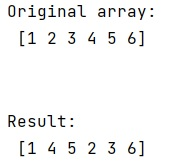# How to swap slices of NumPy arrays?

Learn, how to swap slices of NumPy arrays in Python? By Pranit Sharma Last updated : March 30, 2023

Suppose that we are given a numpy array and we are performing some operation on this array for which we need to slice this array and swap two slices with each other.

For example, if we are given an array [1,2,3,4,5,6] and we need to swap arr[1:3] with arr[3:5], it will give the result as [1,4,5,2,3,6].

## Swapping slices of NumPy arrays

To swap slices of NumPy arrays, we need an additional variable so that the copy of the slice can be stored in a buffer during the swapping. Once a slice is stored in a buffer, we can assign this slice with another slice and the other slice will be assigned as a copy of the first slice.

Let us understand with the help of an example,

## Python code to swap slices of NumPy arrays

```# Import numpy
import numpy as np

# Creating a numpy array
arr = np.array([1,2,3,4,5,6])

# Display Original array
print("Original array:\n",arr,"\n\n")

# Swapping slices
arr[1:3] , arr[3:5] = arr[3:5],arr[1:3].copy()

# Display result
print("Result:\n",arr)
```

### Output